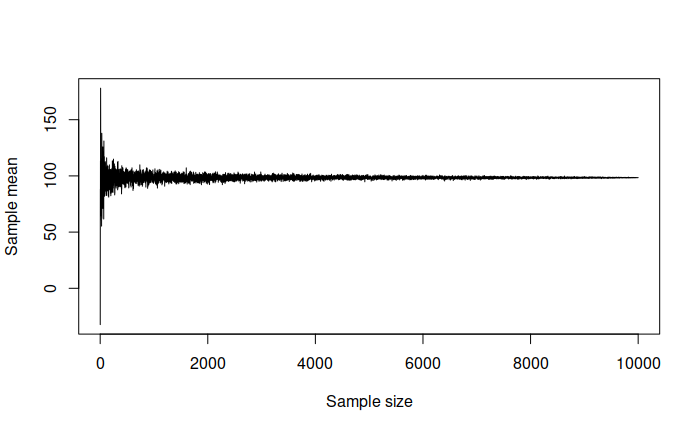This book is in Open Review. I want your feedback to make the book better for you and other readers. To add your annotation, select some text and then click the on the pop-up menu. To see the annotations of others, click the button in the upper right hand corner of the page

## 6.1 Law of Large Numbers

The first law is called the Law of Large Numbers (LLN). It is the theorem saying that (under wide conditions) the average of a variable obtained over the large number of trials will be close to its expected value and will get closer to it with the increase of the sample size. This can be demonstrated with the following example:

obs <- 10000
# Generate data from normal distribution
y <- rnorm(obs,100,100)
# Create sub-samples of 50 and 100 observations
y30 <- sample(y, 30)
y1000 <- sample(y, 1000)
par(mfcol=c(1,2))
hist(y30, xlab="y")
abline(v=mean(y30), col="red")
hist(y1000, xlab="y")
abline(v=mean(y1000), col="red")Figure 6.1: Histograms of samples of data from variable y.

What we will typically see on the plots above is that the mean (red line) on the left plot will be further away from the true mean of 100 than in the case of the right plot. Given that this is randomly generated, the situation might differ, but the idea would be that with the increase of the sample size the estimated sample mean will converge to the true one. We can even produce a plot showing how this happens:

yMean <- vector("numeric",obs)
for(i in 1:obs){
yMean[i] <- mean(sample(y,i))
}
plot(yMean, type="l", xlab="Sample size", ylab="Sample mean")Figure 6.2: Demonstration of Law of Large Numbers.

We can see from the plot above that with the increase of the sample size the sample mean reaches the true value of 100. This is a graphical demonstration of the Law of Large Numbers: it only tells us about what will happen when the sample size increases. But it is still useful, because it used for many statistical inferences and if it does not work, then the estimate of mean would be incorrect, meaning that we cannot make conclusions about the behaviour in population.

In order for LLN to work, the distribution of variable needs to have finite mean and variance. This is discussed in some detail in the next subsection.

In summary, what LLN tells us is that if we average things out over a large number of observations, then that average starts looking very similar to the population value. However, this does not say anything about the performance of estimators on small samples.